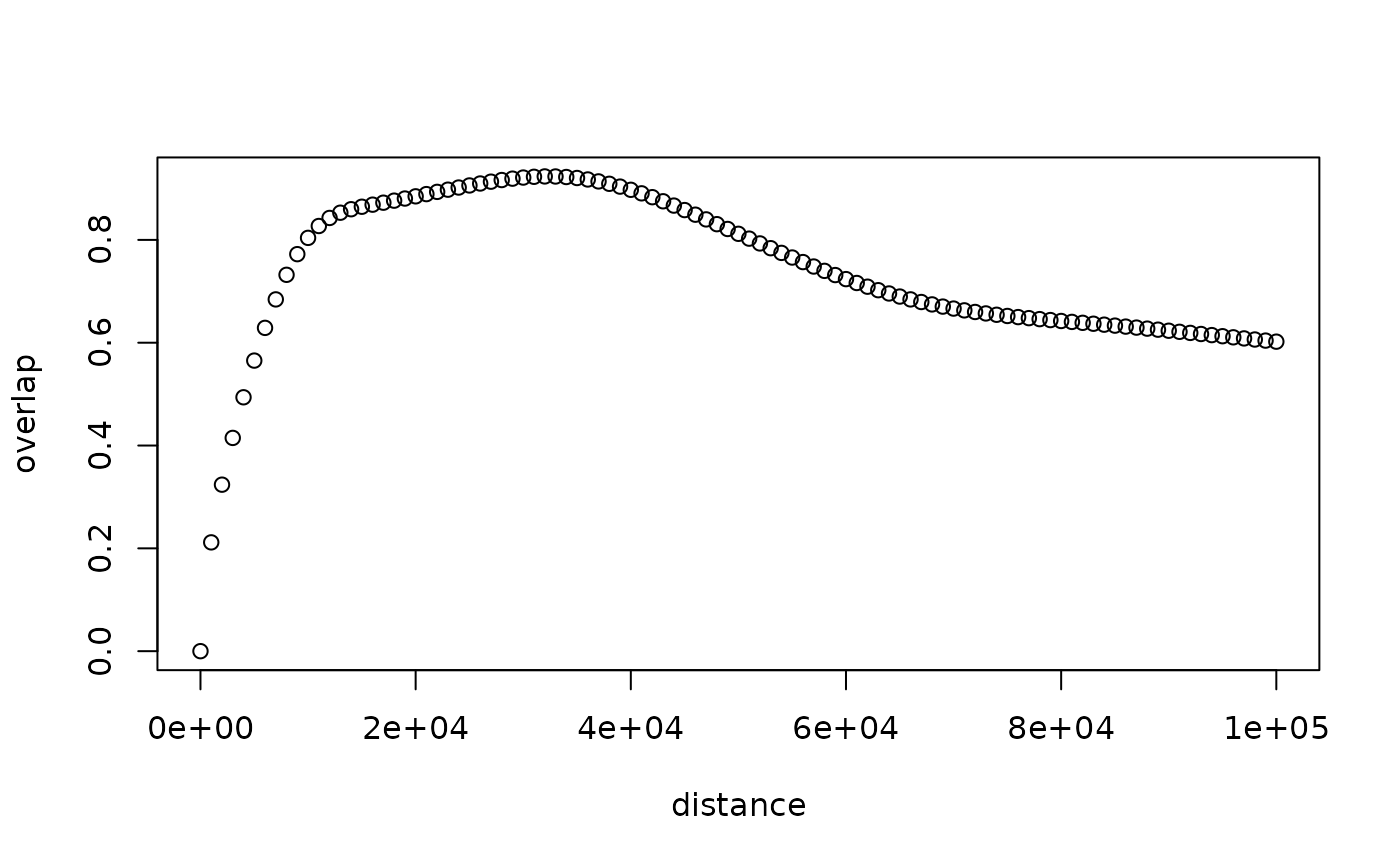Calculates the distribution overlap between a vertical profile ('vp') and the vertical radiation profile of a set of emitted radar beams at various elevation angles as given by beam_profile.

beam_profile_overlap(
vp,
elev,
distance,
antenna,
zlim = c(0, 4000),
noise_floor = -Inf,
noise_floor_ref_range = 1,
steps = 500,
quantity = "dens",
normalize = T,
beam_angle = 1,
k = 4/3,
lat,
re = 6378,
rp = 6357
)

## Arguments

vp

a vertical profile of class vp

elev

numeric vector. Beam elevation(s) in degrees.

distance

the distance(s) from the radar along sea level (down range) for which to calculate the overlap in m.

antenna

radar antenna height. If missing taken from vp

zlim

altitude range in meter, given as a numeric vector of length two.

noise_floor

The system noise floor in dBZ. The total system noise expressed as the reflectivity factor it would represent at a distance noise_floor_ref_range from the radar. NOT YET IMPLEMENTED

noise_floor_ref_range

the reference distance from the radar at which noise_floor is expressed. NOT YET IMPLEMENTED

steps

number of integration steps over altitude range zlim, defining altitude grid size used for numeric integration

quantity

profile quantity to use for the altitude distribution, one of 'dens' or 'eta'.

normalize

Whether to normalize the radiation coverage pattern over the altitude range specified by zlim

beam_angle

numeric. Beam opening angle in degrees, typically the angle between the half-power (-3 dB) points of the main lobe

k

Standard refraction coefficient.

lat

radar latitude. If missing taken from vp

re

rp

## Value

A data.frame with columns distance and overlap.

## Details

This function also calculates the overlap quantity in the output of integrate_to_ppi.

Overlap is calculated as the Bhattacharyya coefficient (i.e. distribution overlap) between the (normalized) vertical profile vp and the (normalized) radiation coverage pattern as calculated by beam_profile. In the calculation of this overlap metric, NA and NaN values in the profile quantity specified by quantity are replaced with zeros.

The current implementation does not (yet) take into account the system noise floor when calculating the overlap.

In the ODIM data model the attribute /how/NEZ or /how/NEZH specifies the system noise floor (the Noise Equivalent Z or noise equivalent reflectivity factor. the H refers to the horizontal channel of a dual-polarization radar). In addition, the attribute /how/LOG gives "security distance above mean noise level (dB) threshold value". This is equivalent to the log receiver signal-to-noise ratio, i.e. the dB above the noise floor for the signal processor to report a valid reflectivity value. We recommend using NEZH+LOG for noise_floor, as this is the effective noise floor of the system below which no data will be reported by the radar signal processor.

Typical values are NEZH = -45 to -50 dBZ at 1 km from the radar. LOG is typically around 1 dB.

Need to evaluate beam by beam the returned signal relative to a uniform beam filling of at least NEZH + LOG If returned signal is lower, the gate is below noise level.

## Examples

# locate example volume file:
pvolfile <- system.file("extdata", "volume.h5", package = "bioRad")

# load the example polar volume file:

# let us use this example vertical profile:
data(example_vp)
example_vp
#>                Vertical profile (class vp)
#>